Courses

# RD Sharma Solutions - Ex-16.2, Congruence, Class 7, Math Class 7 Notes | EduRev

## Class 7: RD Sharma Solutions - Ex-16.2, Congruence, Class 7, Math Class 7 Notes | EduRev

The document RD Sharma Solutions - Ex-16.2, Congruence, Class 7, Math Class 7 Notes | EduRev is a part of the Class 7 Course RD Sharma Solutions for Class 7 Mathematics.
All you need of Class 7 at this link: Class 7

#### Question 1:

In the following pairs of triangles (Fig. 12 to 15), the lengths of the sides are indicated along sides. By applying SSS condition, determine which are congruent. State the result in symbolic form.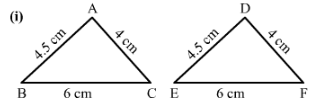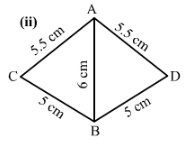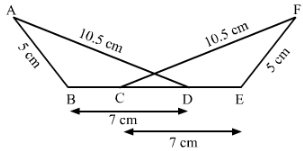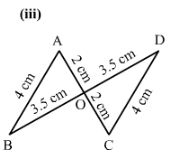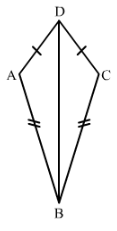1)    In ΔABC and ΔDEF∆ABC and ∆DEF
AB = DE = 4.5 cm (Side)
BC = EF = 6 cm (Side)
and AC = DF = 4 cm (Side)

Therefore, by SSS criterion of congruence, △ABC≅△DEF△ABC≅△DEF.

2)
BC = BD (Side)
and AB=AB (Side)

Therefore, by SSS criterion of congruence, △ACB≅△ADB

3)
In △ABD and △FEC,
AB = FE (Side)
BD = CE  (Side)

Therefore, by SSS criterion of congruence, △ABD≅△FEC△ABD≅△FEC.

#### Question 2:

In Fig. 16, ADDC and ABBC.
(i) Is ∆ ABD ≅ ∆ CBD?
(ii) State the three parts of matching pairs you have used to answer (i).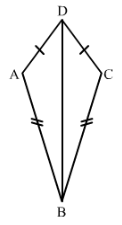Yes △ABD≅△CBD△ABD≅△CBD by the SSS criterion.
We have used the three conditions in the SSS criterion as follows:
AB = BC
and DB = BD

#### Question 3:

In  Fig. 17, ABDC and BCAD.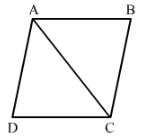(i) Is ∆ ABC ≅ ∆ CDA?
(ii) What congruence condition have you used?
(iii) You have used some fact, not given in the question, what is that?

We have AB = DC
and AC = AC
Therefore by SSS △ABC≅△CDA.

We have used Side Side Side congruence condition with one side common in both the triangles.

Yes, we have used the fact that AC = CA.

#### Question 4:

If ∆ PQR ≅ ∆ EFD,
(i) Which side of ∆ PQR equals ED?
(ii) Which angle of ∆ PQR equals ∠E?

△PQR ≅ △EDF

1) Therefore PR = ED since the corresponding sides of congruent triangles are equal.

2) ∠QPR = ∠FED since the corresponding angles of congruent triangles are equal.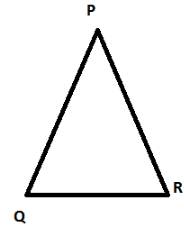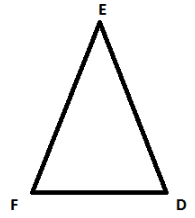#### Question 5:

Triangles ABC and PQR are both isosceles with ABAC and PQPR respectively. If also, ABPQ and BCQR, are the two triangles congruent? Which condition do you use?
If ∠B = 50°, what is the measure of ∠R?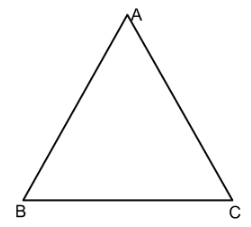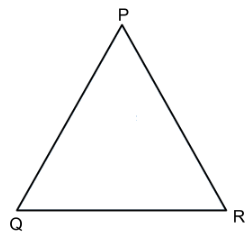We have AB = AC in isosceles △ABC
and PQ = PR in isosceles △PQR.
Also, we are given that AB = PQ and QR = BC.

Therefore, AC = PR (AB = AC, PQ = PR and AB = PQ)
Hence, △ABC≅△PQR.
Now
∠ABC = ∠PQR (Since triangles are congruent)
However, △PQR is isosceles.
Therefore, ∠PRQ =∠PQR =∠ABC = 50°

#### Question 6:

ABC and DBC are both isosceles triangles on a common base BC such that A and D lie on the same side of BC. Are triangles ADB and ADC congruent? Which condition do you use? If ∠BAC = 40° and∠BDC = 100°; then find ∠ADB.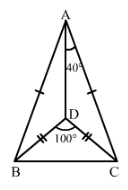AB = AC , DB = DC AND AD= DA

∠ABC+∠BCA+∠BAC=180°  (Angle sum property)
Since ΔABC is an isosceles triangle,
∠ABC=∠BCA
∠ABC+∠ABC+40°=180°
2∠ABC=180°−40°=140°
∠ABC=140°/2=70°

∠DBC+∠BCD+∠BDC=180°  (Angle sum property)
Since ΔABC is an isosceles triangle,
∠DBC=∠BCD
∠DBC+∠DBC+100°=180°
2∠DBC=180°−100°=80°
∠DBC=80°/2=40°

#### Question 7:

∆ ABC and ∆ ABD are on a common base AB, and ACBD and BCAD as shown in Fig. 18. Which of the following statements is true?
(i) ∆ ABC ≅ ∆ ABD
(ii) ∆ ABC ≅ ∆ ADB
(iii) ∆ ABC ≅ ∆ BAD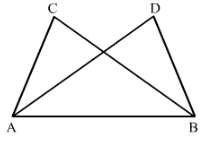In △ABC and △BAD we have,
AC = BD (given)
and AB = BA (common)
Therefore by SSS criterion of congruency, △ABC ≅≅△BAD.
There option (iii) is true.

#### Question 8:

In Fig. 19, ∆ ABC is isosceles with ABACD is the mid-point of base BC.
(ii) State the three pairs of matching parts you use to arrive at your answer.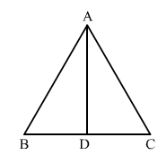We have AB = AC.
Also since D is the midpoint of BC, BD = DC.

We have used AB, AC : BD, DC and AD, DA.

#### Question 9:

In Fig. 20, ∆ ABC is isosceles with ABAC. State if ∆ ABC ≅ ∆ ACB. If yes, state three relations that you use to arrive at your answer.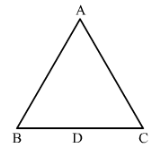Yes △ABC ≅△ACB  by SSS condition.
Since ABC is an isosceles triangle, AB = AC, BC = CB and AC = AB.

#### Question 10:

Triangles ABC and DBC have side BC common, ABBD and ACCD. Are the two triangles congruent? State in symbolic form. Which congruence condition do you use? Does ∠ABD equal ∠ACD? Why or why not?

Yes.

In ΔABC and ΔDB
CAB=DB (Given)
AC=DC (Given)
BC=BC (Common)
By SSS criterion of congruency, ΔABC≅ΔDBC

No, ∠ABD and∠ACD are not equal
because AB ≠ AC.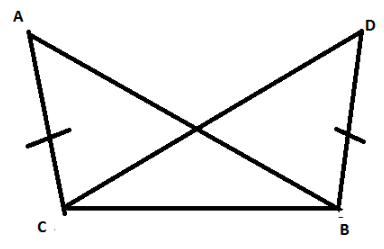The document RD Sharma Solutions - Ex-16.2, Congruence, Class 7, Math Class 7 Notes | EduRev is a part of the Class 7 Course RD Sharma Solutions for Class 7 Mathematics.
All you need of Class 7 at this link: Class 7Use Code STAYHOME200 and get INR 200 additional OFF Use Coupon Code
All Tests, Videos & Notes of Class 7: Class 7

### Top Courses for Class 7## RD Sharma Solutions for Class 7 Mathematics

97 docs

### Top Courses for Class 7Track your progress, build streaks, highlight & save important lessons and more!

,

,

,

,

,

,

,

,

,

,

,

,

,

,

,

,

,

,

,

,

,

,

,

,

,

,

,

,

,

,

;